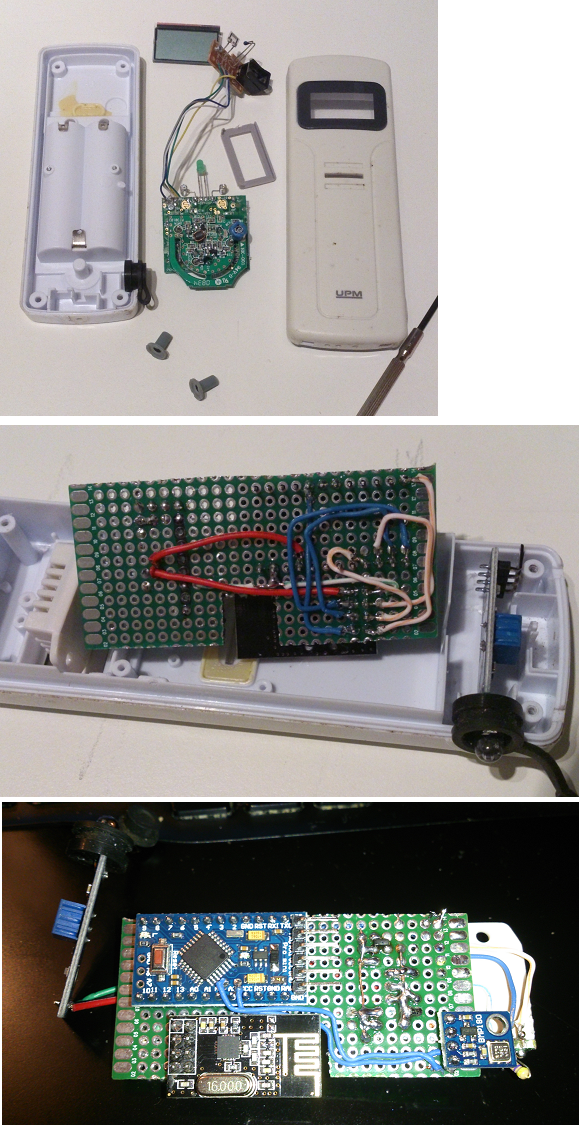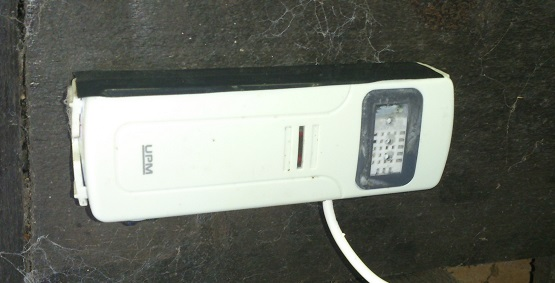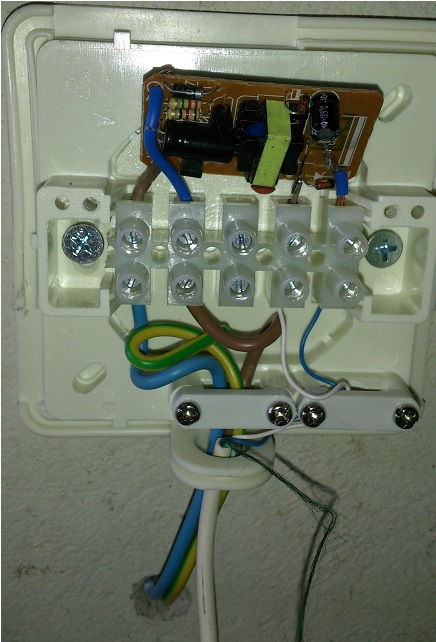# My outdoor weather-sensor (5v powered combined temp, hum, pressure, and light)

• Hello!
My first sensor not powered with battery - a outdoor sensor.

A old temp sensor from a weather station was remade as box.
With some drilling everything fit good inside.

Inside DHT22 (Temp and Hum), LM393 (Light) and BMP085 (Pressure (Temp disabled)).

Some build pictures:and the final result:Everything seems to work perfect in controller... now i can start making some relays to my window lamps so they can power up only when dark enoughThe code as well offcource:

``````#include <SPI.h>
#include <MySensor.h>
#include <Wire.h>
#include <DHT.h>

//Sketch/Node
#define SketchName "Ute sensor x4"
#define SketchVer "1.3"
#define NodeID 5

//Bmp
#define BARO_CHILD 0
#define TEMP_CHILD 1
#define MetersAboveSeaLevel 130
//DHT
#define CHILD_ID_HUM 2
#define CHILD_ID_TEMP 3
#define HUMIDITY_SENSOR_DIGITAL_PIN 3
//Light/LUX
#define CHILD_ID_LIGHT 4
#define LIGHT_SENSOR_ANALOG_PIN 0

unsigned long SLEEP_TIME = 1*60000;
//unsigned long SLEEP_TIME = 10000; //debug

DHT dht;

MyMessage tempMsg(TEMP_CHILD, V_TEMP);
MyMessage pressureMsg(BARO_CHILD, V_PRESSURE);
MyMessage forecastMsg(BARO_CHILD, V_FORECAST);

MyMessage msgHum(CHILD_ID_HUM, V_HUM);
MyMessage msgTemp(CHILD_ID_TEMP, V_TEMP);

MyMessage msg(CHILD_ID_LIGHT, V_LIGHT_LEVEL);

MySensor gw;

//BMP
float lastPressure = -1;
int lastForecast = -1;
int heartbeatBMP = 0;
//float pressureSamples;

const char *weather[] = { "stable", "sunny", "cloudy", "unstable", "thunderstorm", "unknown" };
enum FORECAST
{
STABLE = 0,         // Stable weather
SUNNY = 1,          // Slowly rising HP stable good weather
CLOUDY = 2,         // Slowly falling Low Pressure System, stable rainy weather
UNSTABLE = 3,       // Quickly rising HP, not stable weather
THUNDERSTORM = 4,   // Quickly falling LP, Thunderstorm, not stable
UNKNOWN = 5         // Unknown, more time needed
};

const int LAST_SAMPLES_COUNT = 5;
float lastPressureSamples[LAST_SAMPLES_COUNT];
#define MULTIPLIER (65.0/1023.0)

int minuteCount = 0;
bool firstRound = true;
float pressureAvg;
float dP_dt;
boolean metric;

//DHT
float lastTemp;
float lastHum;

//Light/LUX
int lastLightLevel;
int heartbeatLUX = 0;

void setup() {
gw.begin(NULL, NodeID, false);
//incomingMessageCallback - Callback function for incoming messages from other nodes or controller and request responses. Default is NULL.
//nodeId - The unique id (1-254) for this sensor. Default is AUTO(255) which means sensor tries to fetch an id from controller.
//repeaterMode - Activate repeater mode. This node will forward messages to other nodes in the radio network. Make sure to call process() regularly. Default in false

// Send the sketch version information to the gateway and Controller
gw.sendSketchInfo(SketchName, SketchVer);

if (!bmp.begin()) {
Serial.println("Could not find a valid BMP085 sensor, check wiring!");
while (1) { }
}

//Setup DHT
dht.setup(HUMIDITY_SENSOR_DIGITAL_PIN);

// Register sensors to gw (they will be created as child devices)
gw.present(BARO_CHILD, S_BARO);
gw.present(TEMP_CHILD, S_TEMP);

gw.present(CHILD_ID_HUM, S_HUM);
gw.present(CHILD_ID_TEMP, S_TEMP);

gw.present(CHILD_ID_LIGHT, V_LIGHT_LEVEL);
metric = gw.getConfig().isMetric;
}

void loop() {

//-----------------------------------------------------------------------------//DHT
delay(dht.getMinimumSamplingPeriod());
float temperature = dht.getTemperature();

if (isnan(temperature)) {
} else if (temperature != lastTemp) {
lastTemp = temperature;
if (!metric) {
temperature = dht.toFahrenheit(temperature);
}
gw.send(msgTemp.set(temperature, 1));
Serial.print("T: ");
Serial.println(temperature);
}

float humidity = dht.getHumidity();
if (isnan(humidity)) {
} else if (humidity != lastHum) {
lastHum = humidity;
gw.send(msgHum.set(humidity, 1));
Serial.print("H: ");
Serial.println(humidity);
}

//-----------------------------------------------------------------------------//BPM

float pressure = bmp.readSealevelPressure(MetersAboveSeaLevel)/100; // 130 meters above sealevel
int forecast = sample(pressure);
//Temp fetched from DHT

Serial.print("Temperature = ");
Serial.print(temperature);
Serial.println(metric?" *C":" *F");
Serial.print("Pressure = ");
Serial.print(pressure);
Serial.println(" Pa");
Serial.println(weather[forecast]);

if (isnan(pressure)) {
} else {

if (pressure != lastPressure) {
gw.send(pressureMsg.set(pressure, 0));
lastPressure = pressure;
heartbeatBMP = 0;

}
else
{
heartbeatBMP++;
if (heartbeatBMP >= 24) {

gw.send(pressureMsg.set(pressure, 0));
heartbeatBMP = 0;
}
}
}

if (forecast != lastForecast) {
gw.send(forecastMsg.set(weather[forecast]));
lastForecast = forecast;
}

/*
DP/Dt explanation

0 = "Stable Weather Pattern"
1 = "Slowly rising Good Weather", "Clear/Sunny "
2 = "Slowly falling L-Pressure ", "Cloudy/Rain "
3 = "Quickly rising H-Press",     "Not Stable"
4 = "Quickly falling L-Press",    "Thunderstorm"
5 = "Unknown (More Time needed)
*/
//-----------------------------------------------------------------------------//LJUS

Serial.print("Light: ");
Serial.println(lightLevel);
if (lightLevel != lastLightLevel) {
gw.send(msg.set(lightLevel));
lastLightLevel = lightLevel;
heartbeatLUX = 0;

}
else
{
heartbeatLUX++;
if (heartbeatLUX >= 24) {

gw.send(msg.set(lightLevel));
heartbeatLUX = 0;
}
}

//-----------------------------------------------------------------------------//SLEEP

gw.sleep(SLEEP_TIME); //sleep a bit
}
float getLastPressureSamplesAverage()
{
float lastPressureSamplesAverage = 0;
for (int i = 0; i < LAST_SAMPLES_COUNT; i++)
{
lastPressureSamplesAverage += lastPressureSamples[i];
}
lastPressureSamplesAverage /= LAST_SAMPLES_COUNT;

return lastPressureSamplesAverage ;
}

// Algorithm found here
// http://www.freescale.com/files/sensors/doc/app_note/AN3914.pdf

int sample(float pressure) {

// Calculate the average of the last n minutes.
int index = minuteCount % LAST_SAMPLES_COUNT;
lastPressureSamples[index] = pressure;

minuteCount++;
if (minuteCount > 185)
minuteCount = 6;

if (minuteCount == 5) {
pressureAvg = getLastPressureSamplesAverage();
}
else if (minuteCount == 35) {
pressureAvg = getLastPressureSamplesAverage();
float change = (pressureAvg - pressureAvg);
if (firstRound) // first time initial 3 hour
dP_dt = (MULTIPLIER * 2 * change); // note this is for t = 0.5hour
else
dP_dt = ((MULTIPLIER * change) / 1.5); // divide by 1.5 as this is the difference in time from 0 value.
}
else if (minuteCount == 65) {
pressureAvg = getLastPressureSamplesAverage();
float change = (pressureAvg - pressureAvg);
if (firstRound) //first time initial 3 hour
dP_dt = (MULTIPLIER * change); //note this is for t = 1 hour
else
dP_dt = ((MULTIPLIER * change) / 2); //divide by 2 as this is the difference in time from 0 value
}
else if (minuteCount == 95) {
pressureAvg = getLastPressureSamplesAverage();
float change = (pressureAvg - pressureAvg);
if (firstRound) // first time initial 3 hour
dP_dt = ((MULTIPLIER * change) / 1.5); // note this is for t = 1.5 hour
else
dP_dt = ((MULTIPLIER * change) / 2.5); // divide by 2.5 as this is the difference in time from 0 value
}
else if (minuteCount == 125) {
pressureAvg = getLastPressureSamplesAverage();
float change = (pressureAvg - pressureAvg);
if (firstRound) // first time initial 3 hour
dP_dt = ((MULTIPLIER * change) / 2); // note this is for t = 2 hour
else
dP_dt = ((MULTIPLIER * change) / 3); // divide by 3 as this is the difference in time from 0 value
}
else if (minuteCount == 155) {
pressureAvg = getLastPressureSamplesAverage();
float change = (pressureAvg - pressureAvg);
if (firstRound) // first time initial 3 hour
dP_dt = ((MULTIPLIER * change) / 2.5); // note this is for t = 2.5 hour
else
dP_dt = ((MULTIPLIER * change) / 3.5); // divide by 3.5 as this is the difference in time from 0 value
}
else if (minuteCount == 185) {
pressureAvg = getLastPressureSamplesAverage();
float change = (pressureAvg - pressureAvg);
if (firstRound) // first time initial 3 hour
dP_dt = ((MULTIPLIER * change) / 3); // note this is for t = 3 hour
else
dP_dt = ((MULTIPLIER * change) / 4); // divide by 4 as this is the difference in time from 0 value

pressureAvg = pressureAvg; // Equating the pressure at 0 to the pressure at 2 hour after 3 hours have past.
firstRound = false; // flag to let you know that this is on the past 3 hour mark. Initialized to 0 outside main loop.
}

int forecast = UNKNOWN;
if (minuteCount < 35 && firstRound) //if time is less than 35 min on the first 3 hour interval.
forecast = UNKNOWN;
else if (dP_dt < (-0.25))
forecast = THUNDERSTORM;
else if (dP_dt > 0.25)
forecast = UNSTABLE;
else if ((dP_dt > (-0.25)) && (dP_dt < (-0.05)))
forecast = CLOUDY;
else if ((dP_dt > 0.05) && (dP_dt < 0.25))
forecast = SUNNY;
else if ((dP_dt >(-0.05)) && (dP_dt < 0.05))
forecast = STABLE;
else
forecast = UNKNOWN; // Unknown

return forecast;
}
``````

Edit: Updated the code for right forecast algorithm See here

• @sundberg84
Nice. But don't you miss a gw.process() call in your sketch? It looks like you wanted a repeater node. Just remove the gw.sleep(..) and replace it with gw.process() and some timing if-statement.

• @m26872 Yes i did! - Thank you, good feedback.

Also i will clean upp the code some, because it send temp both from DHT and BMP now i think.

• I ended up not using the process() but sleep() because with three sensors it was spamming the controller.
I thought about some if statements or something to limit the spam but realised that i didnt needed it bad as a relay so...

Also attaching a picture of my power supply - a Ipad plug stripped and used as a 240v -> 5v converter inside a outdoor safe box.Then ran a telephone wire with the 5v to the sensor.

• To make sure outdoor boxed equipment survive a swedish climate more than a year, I'd put some heater inside each box as well. Even a waterproof design will produce moisture from the inside air due to the temperature variations.

• @m26872 I'm interested in what is best against moisture in outside equipment. Would just ventilation be a good idea? Traffic system controllers are not in heated or waterproof casings.. Any experience somebody?

• @AWI @m26872 I've had X-10 indoor rated outlets installed outside in northern Canadian climate for 7+ years with no failures. Humidity was not so much a problem, but extreme temperatures were (-45C to +35C). Their fronts were shielded from direct contact with rain & snow and the outlet itself was in an outdoor rated outlet box, but this was not 100% waterproof nor was it heated other than from the waste heat of the electronics.

Cheers
Al

• I started this thread to continue enclosure protection discussion without polluting this project thread to much.

• ## --- New question on this old post

This node has started to behave strange. There is three sensors and lux is working 100% but DHT and LM393 stops working from time to time. It can suddenly stop sending these two values for 24 hours, and then they are back online again. Lux sensor works all the time.

Havent bothered to do a serial log yet since its outside and working most of the time - but any thoughs anyone?